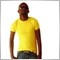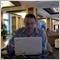# so every time i complete coding i always find my code does not work on the tester. can someone explain to me why this one will not work?35

```//+------------------------------------------------------------------+
//|                                                 Gold Smasher.mq4 |
//|                        Copyright 2017, MetaQuotes Software Corp. |
//|                                             https://www.mql5.com |
//+------------------------------------------------------------------+
#property version   "1.00"
#property strict

extern int takeprofit=2000;
extern int magic= 1212;
extern int stoploss=0;
extern double lots=0.01;
bool did_we_get_refrence_bar=False;
double refrence_high, refrence_low;
int init( )   {GetTickCount();

return(0);}
int deinit(){return(0);}
void OnTick()
{if(DayOfWeek()==1)
{if(did_we_get_refrence_bar==False){
if(is_It_this_time_on_this_bar("09:00",0)==True)
{if (is_the_previous_bar_bigger("09:00",0,300)==False)
{did_we_get_refrence_bar=True;
refrence_high=High;
refrence_low=Low;}}}

if(did_we_get_refrence_bar==False)
{if(is_It_this_time_on_this_bar("13:00",0)==True)
{if (is_the_previous_bar_bigger("13:00",0,300)==False)
{did_we_get_refrence_bar=True;
refrence_high=High;
refrence_low=Low;}}}

if(did_we_get_refrence_bar==False)
{if(is_It_this_time_on_this_bar("17:00",0)==True)
{if (is_the_previous_bar_bigger("17:00",0,300)==False)
{did_we_get_refrence_bar=True;
refrence_high=High;
refrence_low=Low;}}}

if(did_we_get_refrence_bar==False)
{if(is_It_this_time_on_this_bar("21:00",0)==True)
{if (is_the_previous_bar_bigger("21:00",0,300)==False)
{did_we_get_refrence_bar=True;
refrence_high=High;
refrence_low=Low;}}}}

if(DayOfWeek()==2)
{if(did_we_get_refrence_bar==False)
{if(is_It_this_time_on_this_bar("01:00",0)==True)
{if (is_the_previous_bar_bigger("01:00",0,300)==False)
{did_we_get_refrence_bar=True;
refrence_high=High;
refrence_low=Low;}}}

if(did_we_get_refrence_bar==False)
{if(is_It_this_time_on_this_bar("05:00",0)==True)
{if (is_the_previous_bar_bigger("05:00",0,300)==False)
{did_we_get_refrence_bar=True;
refrence_high=High;
refrence_low=Low;}}}

if(did_we_get_refrence_bar==False)
{if(is_It_this_time_on_this_bar("09:00",0)==True)
{if (is_the_previous_bar_bigger("09:00",0,300)==False)
{did_we_get_refrence_bar=True;
refrence_high=High;
refrence_low=Low;}}}

if(did_we_get_refrence_bar==False)
{if(is_It_this_time_on_this_bar("13:00",0)==True)
{if (is_the_previous_bar_bigger("13:00",0,300)==False)
{did_we_get_refrence_bar=True;
refrence_high=High;
refrence_low=Low;}}}

if(did_we_get_refrence_bar==False)
{if(is_It_this_time_on_this_bar("17:00",0)==True)
{if (is_the_previous_bar_bigger("17:00",0,300)==False)
{did_we_get_refrence_bar=True;
refrence_high=High;
refrence_low=Low;}}}

if(did_we_get_refrence_bar==False)
{if(is_It_this_time_on_this_bar("21:00",0)==True)
{if (is_the_previous_bar_bigger("21:00",0,300)==False)
{did_we_get_refrence_bar=True;
refrence_high=High;
refrence_low=Low;}}}}

if(DayOfWeek()==3)
{if(did_we_get_refrence_bar==False)
{if(is_It_this_time_on_this_bar("01:00",0)==True)
{if (is_the_previous_bar_bigger("01:00",0,300)==False)
{did_we_get_refrence_bar=True;
refrence_high=High;
refrence_low=Low;}}}

if(did_we_get_refrence_bar==False)
{if(is_It_this_time_on_this_bar("05:00",0)==True)
{if (is_the_previous_bar_bigger("05:00",0,300)==False)
{did_we_get_refrence_bar=True;
refrence_high=High;
refrence_low=Low;}}}

if(did_we_get_refrence_bar==False)
{ if(is_It_this_time_on_this_bar("09:00",0)==True)
{if (is_the_previous_bar_bigger("09:00",0,300)==False)
{did_we_get_refrence_bar=True;
refrence_high=High;
refrence_low=Low;}}}

if(did_we_get_refrence_bar==False){
if(is_It_this_time_on_this_bar("13:00",0)==True)
{if (is_the_previous_bar_bigger("13:00",0,300)==False)
{did_we_get_refrence_bar=True;
refrence_high=High;
refrence_low=Low;}}}

if(did_we_get_refrence_bar==False){
if(is_It_this_time_on_this_bar("17:00",0)==True)
{if (is_the_previous_bar_bigger("17:00",0,300)==False)
{did_we_get_refrence_bar=True;
refrence_high=High;
refrence_low=Low;}}}
if(did_we_get_refrence_bar==False)
{if(is_It_this_time_on_this_bar("21:00",0)==True)
{if (is_the_previous_bar_bigger("21:00",0,300)==False)
{did_we_get_refrence_bar=True;
refrence_high=High;
refrence_low=Low;}}}}

if(did_we_get_refrence_bar==True)                   //once a refrence bar is chosen.
{if(Close>refrence_high)               //if the previous bar closed above our refrence bar.
{if(Close<=refrence_high)              // the bar before the bar that closed above refrence high should have been lower than the high.
if (sells()>0){closePosition(OP_SELL);}}}}

if(Close<refrence_low)
{if(Close>=refrence_low)
{if(sells()<0)
{openPosition(OP_SELL);

if(DayOfWeek()==5)                                              //used for the final day of the week to close all open positions.
{if(is_It_this_time_on_this_bar("21:00",0)==True)
{did_we_get_refrence_bar=False;                    // reset all parameters for the following week.
OnTick();                                          //points towards the start operatons.
}}}

void openPosition(int tradeType)                 //opening positions function.
{double tp, price;
color onColor;
onColor = clrLimeGreen;
tp = Bid + takeprofit * Point;}
else{ price = Bid;
onColor = clrRed;
tp = Ask - takeprofit * Point;}
if (OrderSend(Symbol(), tradeType, lots, price, 3,0 , tp, "", magic, 0, onColor) == -1)
{GetLastError();}}

void closePosition(int tradeType)               // closing positions funtion.
{double price;
for (int i = OrdersTotal() - 1; i >= 0; i--)
{if (OrderSymbol() == Symbol() && OrderMagicNumber() == magic && OrderType() == tradeType)
{price = Bid;}
else
if (!OrderClose(OrderTicket(), OrderLots(), price, 3, clrWhite)) GetLastError();}}}

bool is_It_this_time_on_this_bar(string time,int x)    // int x take the bar number(zero for current bar), time takes a tring of time e.g "00:00".
{datetime candle_time=Time[x];
string candle_time_string=TimeToStr(candle_time,TIME_MINUTES);
bool true_or_false=False;
if(candle_time_string==time){true_or_false=True;}
return(true_or_false);}        // gives you a true value if the bar number is at the input time.

bool is_the_previous_bar_bigger(string time,int x, double points)    // int x take the bar number(zero for current bar), time takes a tring of time e.g "00:00".
{datetime candle_time=Time[x];
string candle_time_string=TimeToStr(candle_time,TIME_MINUTES);
bool true_or_false=False;
if((High[x+1]-Low[x+1])>(points*Point))
{true_or_false=True;}
return(true_or_false);}        // gives you a true value if the bar previous bar is bigger tha the points inputs.

int sells()          //it take the magic of the EA and give the total sells under the EA.
int sells = 0;
for (int i = 0; i < OrdersTotal(); i++)
{if (OrderSelect(i, SELECT_BY_POS))
{if (OrderSymbol() != Symbol() && OrderMagicNumber() != magic) continue;
else
{if (OrderType() == OP_SELL)
{sells++;}}}}
return(sells);}

int buys()          //it take the magic of the EA and give the total buys under the EA.
int sells = 0;
for (int i = 0; i < OrdersTotal(); i++)
{if (OrderSelect(i, SELECT_BY_POS))
{if (OrderSymbol() != Symbol() && OrderMagicNumber() != magic) continue;
else
{if (OrderType() == OP_SELL)
{sells++;}}}}

here is the code.• www.mql5.com
Choose a suitable trading strategy and subscribe to it with a few clicks. All Signals are provided with detailed statistics and informative charts. Become a trading signal provider and sell subscriptions to thousands of traders around the world. With the Signals service, your successful strategy can generate income with a small start-up budget...Moderator
12341125824

2. `if (OrderSymbol() != Symbol() && OrderMagicNumber() != magic) continue;`
3. ``` bool true_or_false=False;
4. `if(did_we_get_refrence_bar==True)`